### K Means Clustering in Machine Learning

• date 15th February, 2020 |
• by Prwatech |

# K Means Clustering in Machine Learning

K Means Clustering in Machine Learning, in this Tutorial one can learn k-means clustering introduction. Are you the one who is looking for the best platform which provides information about k means clustering algorithm steps,advantages of k means clustering algorithm, k means clustering algorithm example? Or the one who is looking forward to taking the advanced Data Science Certification Course with Machine Learning from India’s Leading Data Science Training institute? Then you’ve landed on the Right Path.

K-means clustering is one among the best and popular unsupervised machine learning algorithms. during this blog, we are going to understand the K-Means clustering algorithm with the assistance of examples.Before aiming to K-means Clustering, allow us to first understand what Clustering is.Clustering helps to group similar data points together while these groups are significantly different from one another. K-means clustering is one among the best and popular unsupervised machine learning algorithms.

The Below mentioned Tutorial will help to Understand the detailed information about k means clustering in machine learning, so Just follow all the tutorials of India’s Leading Best Data Science Training institute in Bangalore and Be a Pro Data Scientist or Machine Learning Engineer.

## K Means Clustering Introduction

Unsupervised algorithms read datasets using input vectors only, without denoting known or labeled outputs. Means there is no any clear identification of any label or category. One of the most popular unsupervised machine algorithms is K Means Clustering. It can be stated as task of identifying different groups in given unstructured data having similar data points. The meaning of cluster is nothing but subgroups of data points. Clustering assists us to understand our data by grouping things together into clusters.

While dividing data into different clusters it is necessary to understand properties of clusters. All the data points in single cluster should be alike to each other. Also, the data points from different clusters should be as dissimilar as possible.

Some real time examples of using this type of machine learning algorithm are, Identifying Fake News, Spam filter, Marketing and Sales, Classifying network traffic, Identifying fraudulent or criminal activity, document analysis, recommendation engines etc.

## K Means Clustering Algorithm Steps

The algorithm works in following manner:

### Step 1: Choosing the number of clusters k:

Initialize k points, which are nothing but means of clusters.The entity ‘k’ is called means, because they are the mean values of the data points categorized in it indirectly, we are choosing number of clusters. For example, let’s choose 2 clusters, so k is equal to 2

### Step 2: Selecting k random points as centroids from data set:

Here we have to select the mean or centroid for each cluster randomly. Let’s select centroid or means randomly.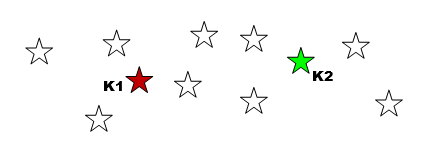### Step 3: Grouping all the points nearest to mean or centroid

Categorize each data point to its nearest mean.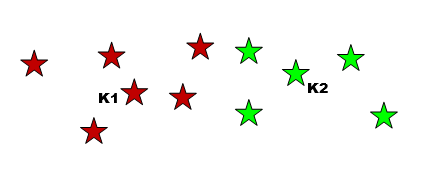### Step 4: Recompute the means of new clusters

Here we have to update the mean’s coordinates, which are the averages of the data points categorized in that group.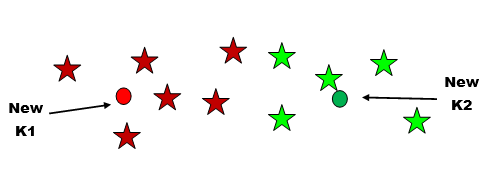### Step 5: Repeat steps 3 and 4

Repeat the process till means will not be changed longer i.e. iterations to be done till we get next means same as previous. Then we get final clusters.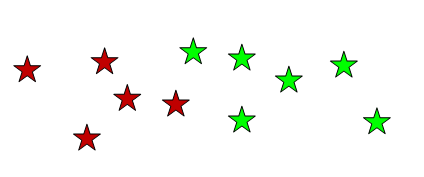What should be the ‘K’ value that will depend on the data set and the problem which we are going to solve.

## Advantages of K Means Clustering Algorithm

Some advantages of K-Means clustering method are:

It is easy to implement

Comparatively speedy and efficient

It has only single parameter to vary and the direct impact of adjusting the value of parameter K can be observed easily.

## K Means Clustering Algorithm Example

Now let’ see example of K Mean Clustering using Python

### Initializing and importing libraries

import numpy as np

import pandas as pd

%matplotlib inline

import matplotlib.pyplot as plt

import seaborn as sns; sns.set()

### Generating set of data points with make_blob

from sklearn.datasets.samples_generator import make_blobs

X, y = make_blobs(n_samples=500, centers=3,cluster_std=0.50, random_state=0)

plt.scatter(X[:, 0], X[:, 1], s=40)

It will show scatter plot as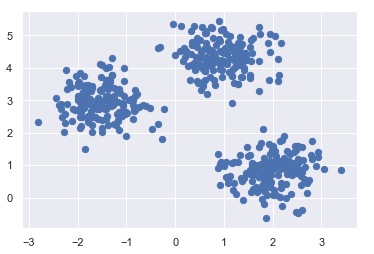### Applying K Means to dataset

from sklearn.cluster import KMeans

kmeans = KMeans(n_clusters=6)  # We can change n_cluster number asper need

kmeans.fit(X)

y_kmeans = kmeans.predict(X)

### Let’s code for visualization for this clustering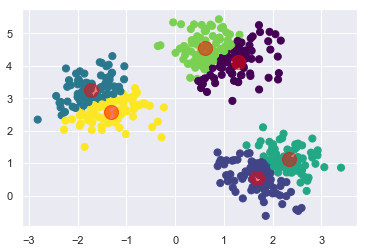plt.scatter(X[:, 0], X[:, 1], c=y_kmeans, s=50, cmap=’viridis’)

cent = kmeans.cluster_centers_

plt.scatter(cent [:, 0], centers[:, 1], c=’red’, s=200, alpha=0.5);

We hope you understand random forest tutorial for beginners.Get success in your career as a Data Scientist by being a part of the Prwatech, India’s leading Data Science training institute in Bangalore.

### Quick Support